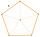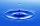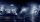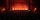# Fraction calculator

The calculator performs basic and advanced operations with fractions, expressions with fractions combined with integers, decimals, and mixed numbers. It also shows detailed step-by-step information about the fraction calculation procedure. Solve problems with two, three, or more fractions and numbers in one expression.

## Result:

### 21/3 + 13/4 = 49/12 = 4 1/12 ≅ 4.0833333

Spelled result in words is forty-nine twelfths (or four and one twelfth).

### How do you solve fractions step by step?

1. Conversion a mixed number 2 1/3 to a improper fraction: 2 1/3 = 2 1/3 = 2 · 3 + 1/3 = 6 + 1/3 = 7/3

To find new numerator:
a) Multiply the whole number 2 by the denominator 3. Whole number 2 equally 2 * 3/3 = 6/3
b) Add the answer from previous step 6 to the numerator 1. New numerator is 6 + 1 = 7
c) Write a previous answer (new numerator 7) over the denominator 3.

Two and one third is seven thirds
2. Conversion a mixed number 1 3/4 to a improper fraction: 1 3/4 = 1 3/4 = 1 · 4 + 3/4 = 4 + 3/4 = 7/4

To find new numerator:
a) Multiply the whole number 1 by the denominator 4. Whole number 1 equally 1 * 4/4 = 4/4
b) Add the answer from previous step 4 to the numerator 3. New numerator is 4 + 3 = 7
c) Write a previous answer (new numerator 7) over the denominator 4.

One and three quarters is seven quarters
3. Add: 7/3 + 7/4 = 7 · 4/3 · 4 + 7 · 3/4 · 3 = 28/12 + 21/12 = 28 + 21/12 = 49/12
For adding, subtracting, and comparing fractions, it is suitable to adjust both fractions to a common (equal, identical) denominator. The common denominator you can calculate as the least common multiple of both denominators - LCM(3, 4) = 12. In practice, it is enough to find the common denominator (not necessarily the lowest) by multiplying the denominators: 3 × 4 = 12. In the next intermediate step, the fraction result cannot be further simplified by canceling.
In words - seven thirds plus seven quarters = forty-nine twelfths.

#### Rules for expressions with fractions:

Fractions - use the slash “/” between the numerator and denominator, i.e., for five-hundredths, enter 5/100. If you are using mixed numbers, be sure to leave a single space between the whole and fraction part.
The slash separates the numerator (number above a fraction line) and denominator (number below).

Mixed numerals (mixed fractions or mixed numbers) write as non-zero integer separated by one space and fraction i.e., 1 2/3 (having the same sign). An example of a negative mixed fraction: -5 1/2.
Because slash is both signs for fraction line and division, we recommended use colon (:) as the operator of division fractions i.e., 1/2 : 3.

Decimals (decimal numbers) enter with a decimal point . and they are automatically converted to fractions - i.e. 1.45.

The colon : and slash / is the symbol of division. Can be used to divide mixed numbers 1 2/3 : 4 3/8 or can be used for write complex fractions i.e. 1/2 : 1/3.
An asterisk * or × is the symbol for multiplication.
Plus + is addition, minus sign - is subtraction and ()[] is mathematical parentheses.
The exponentiation/power symbol is ^ - for example: (7/8-4/5)^2 = (7/8-4/5)2

#### Examples:

subtracting fractions: 2/3 - 1/2
multiplying fractions: 7/8 * 3/9
dividing Fractions: 1/2 : 3/4
exponentiation of fraction: 3/5^3
fractional exponents: 16 ^ 1/2
adding fractions and mixed numbers: 8/5 + 6 2/7
dividing integer and fraction: 5 ÷ 1/2
complex fractions: 5/8 : 2 2/3
decimal to fraction: 0.625
Fraction to Decimal: 1/4
Fraction to Percent: 1/8 %
comparing fractions: 1/4 2/3
multiplying a fraction by a whole number: 6 * 3/4
square root of a fraction: sqrt(1/16)
reducing or simplifying the fraction (simplification) - dividing the numerator and denominator of a fraction by the same non-zero number - equivalent fraction: 4/22
expression with brackets: 1/3 * (1/2 - 3 3/8)
compound fraction: 3/4 of 5/7
fractions multiple: 2/3 of 3/5
divide to find the quotient: 3/5 ÷ 2/3

The calculator follows well-known rules for order of operations. The most common mnemonics for remembering this order of operations are:
PEMDAS - Parentheses, Exponents, Multiplication, Division, Addition, Subtraction.
BEDMAS - Brackets, Exponents, Division, Multiplication, Addition, Subtraction
BODMAS - Brackets, Of or Order, Division, Multiplication, Addition, Subtraction.
GEMDAS - Grouping Symbols - brackets (){}, Exponents, Multiplication, Division, Addition, Subtraction.
Be careful, always do multiplication and division before addition and subtraction. Some operators (+ and -) and (* and /) has the same priority and then must evaluate from left to right.

## Fractions in word problems:

• ExpressionsLet k represent an unknown number, express the following expressions: 1. The sum of the number n and two 2. The quotient of the numbers n and nine 3. Twice the number n 4. The difference between nine and the number n 5. Nine less than the number n
• Of the 2Of the shapes,1/6 are triangles and 5/12 are pentagons. What fraction of the shapes are either triangles or pentagons?
• Math homeworkIt took Jose two-thirds of an hour to complete his math homework on Monday, three-fourths of an hour on Tuesday, any two- fifths of an hour on Wednesday. How many hours did it take Jose to complete his homework altogether?
• EvaluateThe division of numbers \$a and \$b increase by-product of the numbers \$c and \$d
• A shopkeeper 3A shopkeeper sells 8 1/3 kg, 10 1/4 kg and 11 1/5 kg of apples on 3 consecutive days. What is the total weight of apples sold?
• Bathroom 4Dolor puts 3 1/2 pails of water into a water container in the bathroom every day. Her daughter, Lei, uses 2 1/4 pails of water every day when taking a bath. If the water container had 5 5/8 pails of water at the start, how much water is left in it after 5
• The MayflowerThe Mayflower traveled for 66 days on the trip from England to America. The weather was storming for many days of their trip. If one and a half of the days at Sea where Sunny with good weather, 1/6 of the days were sunny but very windy and the other days
• Berry SmoothieRory has 5/8 cup of milk. How much milk does she have left after she doubles the recipe of the smoothie? Berry Smoothie: 2 cups strawberries 1 cup blueberries 1/4 cup milk 1 tbsp (tablespoon) sugar 1/2 tsp (teaspoon) lemon juice 1/8 tsp (teaspoon) vanillaThe weight of the empty fruit basket is ¾ kg. We added ½ kg apples and 5/4 kg guavas. What will be the total weight of the fruit basket?Ann ate a third of a pizza and then another quater. Total part of pizza eaten by Ann and how much pizza is left?Jacobs mom bought two whole pizzas. He ate 2/10 of the pizza and his dad ate 1 1/5. How much is left.A large popcorn bag holds four times as much as a small popcorn bag at the end of the party 3 1/3 small bags and 2 1/4 large bags left. How many small bags with the leftover popcorn fill?Anya, Jose, Cali, and Stephan walk for exercise. Anya's route is 2 1/4 kilometers long. Jose's route is 1 1/2 fewer km. Cali's route is 1 1/2  times as long as Jose's route, and 2 fewer km than Stephan's route. What distance (S) is Stephan's route?